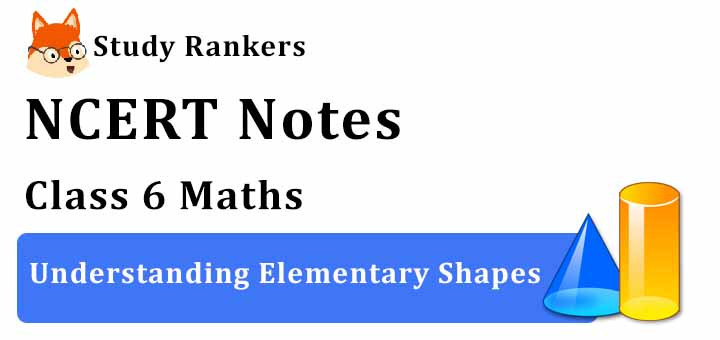# Chapter 5 Understanding Elementary Shapes Class 6 Notes Maths

Chapter 5 Understanding Elementary Shapes Class 6 Notes Maths is available on this page will provide a quick glimpse of the chapter and improve the learning experience. A student will enjoy the revising process and make themselves capable of retaining more information so they can excel in the exams. Through the help of Revision Notes for Class 6, will ensure that remembering and retaining the syllabus more easy and efficient. Also, NCERT Solutions for Class 6 Chapter 5 Maths that will help in completing homework on time.Measuring Line Segments

• A line segment is a fixed portion of a line.

• The distance between two end points of a line segment is called its length.

• A ruler and divider are useful to compare lengths of line segments.

Angles – ‘Right’ and ‘Straight’

• The figure formed by two rays with the same initial point.

• One full turn of the hand = 1 revolution.

• A right angle = 14 revolution.

• A straight angle = 12 revolution.

• We use protractor to measure the size of an angle in degrees.

• Acute angle : An angle whose measure is more than 0° but less than 90°.

• Right angle: An angle whose measure is 90°.

• Obtuse angle: An angle whose measure is more than 90° but less than 180°.

• Straight angle : An angle whose measure is 180°.

• Reflex angle : An angle whose measure is more than 180° but less than 360° is called a reflex angle.

• Complete angle : An angle whose measure is 360°, is called a complete angle.

Perpendicular Lines

• When two lines intersect and the angle between them is a right angle, then the lines are said to be perpendicular. If a line AB is perpendicular to CD, we write AB ⊥ CD.

Classification of Triangles

Types of Triangles based on Sides

(i) Equilateral triangle: A triangle having all sides equal, is called an equilateral triangle.
(ii) Isosceles triangle: A triangle having two sides equal, is called an isosceles triangle.
(iii) Scalene triangle: A triangle having all sides of different lengths is called a scalene triangle.

Types of Triangles based on Angles

(i) Acute triangle: A triangle each of whose angle measures less than 90° is called an acute triangle.
(ii) Right angled triangle: A triangle one of whose angle measures 90° is called a right angled triangle.
(iii) Obtuse triangle: A triangle one of whose angle measures more than 90° is called an obtuse triangle.

Polygons

Polygon is a closed figure bounded by three or more than three line segments is called a polygon.

• If a polygon is bounded by 3 sides, it is called a triangle.

• If a polygon is bounded by 4 sides, it is called a quadrilateral.

• If a polygon is bounded by 5 sides, it is called a pentagon.

• If a polygon is bounded by 6 sides, it is called a hexagon.

• It is a plane figure bounded by four line segments is called a quadrilateral.

• A quadrilateral having opposite pair of sides parallel is called a parallelogram.

• A quadrilateral having only one pair of parallel sides is called a trapezium.

• A quadrilateral having opposite sides equal and each angle of 90° is called rectangle.

• If a quadrilateral is having all sides equal and each angle of 90°, it is called a square.

• A parallelogram having all of its sides equal is called a rhombus.

Three dimensional shape

• Sphere : An object which is in the shape of a ball. It has a curved surface but no vertex and no edge.

• Cone : Objects in the form of ice-cream cone, a conical tent, birthday cap etc.

• Cylinder : A circular pipe, a circular pillar etc. are in the shape of cylinder. It has one curved surface and two circular faces at its ends.

• Cuboid : A brick, a matchbox, a book, etc. are in the shape of a cuboid. It has length, breadth and height. It has 6 rectangular faces. Two faces meet at a line segment called an edge. Three edges meet at a point called vertex.

• Cube : A cuboid whose length breadth and height are equal e.g., dice, ice cubes etc. A cube has 6 square faces, 12 edges and 8 vertices.

• Pyramid : A solid whose base in a plane rectilinear figure and whose side faces are triangles having a common vertex.